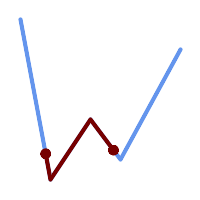## Name

ST_LineSubstring — Return a linestring being a substring of the input one starting and ending at the given fractions of total 2d length. Second and third arguments are float8 values between 0 and 1.

## Synopsis

`geometry ST_LineSubstring(`geometry a_linestring, float8 startfraction, float8 endfraction`)`;

## Description

Return a linestring being a substring of the input one starting and ending at the given fractions of total 2d length. Second and third arguments are float8 values between 0 and 1. This only works with LINESTRINGs. To use with contiguous MULTILINESTRINGs use in conjunction with ST_LineMerge.

If 'start' and 'end' have the same value this is equivalent to ST_LineInterpolatePoint.

See ST_LineLocatePoint for computing the line location nearest to a Point.Since release 1.1.1 this function also interpolates M and Z values (when present), while prior releases set them to unspecified values.

Availability: 1.1.0, Z and M supported added in 1.1.1

Changed: 2.1.0. Up to 2.0.x this was called ST_Line_Substring.This function supports 3d and will not drop the z-index.

## ExamplesA linestring seen with 1/3 midrange overlaid (0.333, 0.666)

```--Return the approximate 1/3 mid-range part of a linestring
SELECT ST_AsText(ST_Line_SubString(ST_GeomFromText('LINESTRING(25 50, 100 125, 150 190)'), 0.333, 0.666));

st_astext
------------------------------------------------------------------------------------------------
LINESTRING(69.2846934853974 94.2846934853974,100 125,111.700356260683 140.210463138888)

--The below example simulates a while loop in
--SQL using PostgreSQL generate_series() to cut all
--linestrings in a table to 100 unit segments
-- of which no segment is longer than 100 units
-- units are measured in the SRID units of measurement
-- It also assumes all geometries are LINESTRING or contiguous MULTILINESTRING
--and no geometry is longer than 100 units*10000
--for better performance you can reduce the 10000
--to match max number of segments you expect

SELECT field1, field2, ST_LineSubstring(the_geom, 100.00*n/length,
CASE
WHEN 100.00*(n+1) < length THEN 100.00*(n+1)/length
ELSE 1
END) As the_geom
FROM
(SELECT sometable.field1, sometable.field2,
ST_LineMerge(sometable.the_geom) AS the_geom,
ST_Length(sometable.the_geom) As length
FROM sometable
) AS t
CROSS JOIN generate_series(0,10000) AS n
WHERE n*100.00/length < 1;
```